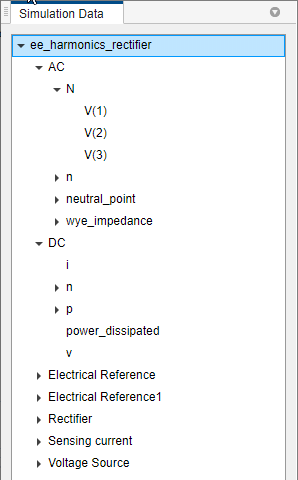# Harmonic Analyzer

Analyze and plot harmonic spectrum of Simscape logging nodes

Since R2022a

## Description

The Harmonic Analyzer app calculates the harmonic orders, magnitudes, and fundamental frequency of a `simscape.logging.Node` of an AC or periodic variable and plots a bar chart of the percentage of fundamental magnitude versus harmonic order.

The Harmonic Analyzer app allows you to:

• Import the simulation data of a model stored in a `simscape.logging.Node` variable.

• Analyze and plot the harmonic spectrum of the selected `simscape.logging.Node` object.

• Export the data in MATLAB® as a script or a function.

The app finds the points in the selected leaf node, or a node at the very end of the tree navigation, where the Simscape™ log crosses a threshold specified by the DC offset parameter. It then uses the crossing points to find the required number of periods, specified by the Number of periods parameter, preceding the value specified by the Simulation time (s) parameter. Finally it inputs the selected data to the Goertzel algorithm, which calculates the harmonic magnitudes up to the value in the Harmonic order parameter.

## Open the Harmonic Analyzer App

To open the app, at the MATLAB Command Window, enter: `ee_HarmonicAnalyzer`.

## Examples

expand all

Open the model. At the MATLAB command prompt, enter:

`ee_harmonics_rectifier`

Run the model. In the Simulation tab, click the Run button.

The base workspace now contains the `simlog_ee_harmonics_rectifier` `Node` object.

Open the Harmonic Analyzer app by entering:

`ee_HarmonicAnalyzer`

To load the simulation data variable of the `ee_harmonics_rectifier` model, in the app, click Import.

In the Simulation data dialog, set Inputs to the `simlog_ee_harmonics_rectifier` `Node` object to import from the base workspace.The app imports all Simscape logging nodes contained in the `simlog_ee_harmonics_rectifier` `Node` object.To analyze and plot the signal and the harmonic spectrum of a logging node, open the Harmonic Analyzer app, click the Simulation Data tab, then select a node. You can only plot the harmonic spectrum of a leaf node.

Follow the steps in Load Simulation Data Variable for the Current Model to open the model, open the app, and load the `Node` object for `simlog_ee_harmonics_rectifier`.

Then select the V(2) node to plot the `b`-phase of the AC node in the Signal Plot tab, as well as its harmonic spectrum in the Harmonic Plot tab.The Panned Signal Plot tab plots the panned signal. Drag the panner in the Signal Plot tab to interactively focus on a specific part of the signal. To increase or decrease the size of the panner, specify the Number of periods parameter.

To export the MATLAB commands required to plot the harmonic spectrum of the selected node outside of the Harmonic Analyzer app, click the Export button and then Script or Function to generate a script or a function, respectively.

## Parameters

expand all

Number of periods of fundamental frequency in the harmonic analysis. The value of this parameter also affects the size of the panner box in the Signal Plot tab.

DC offset in the input signal. The app uses this value to find the periods of interest.

Number of harmonics to include in the analysis.

Time of the last period panned to compute the harmonic spectrum. The final permissible simulation time is equal to the simulation time specified in the model.

## Limitations

The Harmonic Analyzer app does not support `simscape.logging.Node` objects generated by variable step size models.

## Version History

Introduced in R2022a